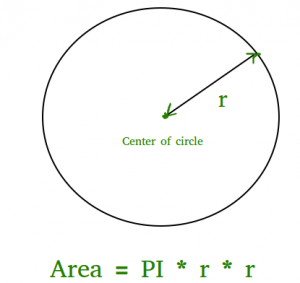# Program to find area of a circle

• Difficulty Level : Medium
• Last Updated : 06 Nov, 2021

The area of a circle can simply be evaluated using the following formula.Hey! Looking for some great resources suitable for young ones? You've come to the right place. Check out our self-paced courses designed for students of grades I-XII

Start with topics like Python, HTML, ML, and learn to make some games and apps all with the help of our expertly designed content! So students worry no more, because GeeksforGeeks School is now here!

```Area = pi * r2
where r is radius of circle and it maybe in float because value of pie is 3.14 ```

## C++

 `// C++ program to find area``// of circle``#include ``const` `double` `pi = 3.14159265358979323846;``using` `namespace` `std;` `// function to calculate the area of circle``float` `findArea(``float` `r)``{``    ``return` `(pi * r * r);``}``// driver code``int` `main()``{``    ``float` `r, Area;``    ``r = 5;` `    ``// function calling``    ``Area = findArea(r);``    ``// displaying the area``    ``cout << ``"Area of Circle is :"` `<< Area;``    ``return` `0;``}`

## C

 `// C program to find area``// of circle``#include ``#include ``#define PI 3.142` `double` `findArea(``int` `r)``{``   ``return` `PI * ``pow``(r, 2);``}` `int` `main()``{``    ``printf``(``"Area is %f"``, findArea(5));``    ``return` `0;``}`

## Java

 `// Java program to find area``// of circle``    ` `class` `Test``{``    ``static` `final` `double` `PI = Math.PI;``         ` `    ``static` `double` `findArea(``int` `r)``    ``{``       ``return` `PI * Math.pow(r, ``2``);``    ``}``         ` `    ``// Driver method``    ``public` `static` `void` `main(String[] args)``    ``{``        ``System.out.println(``"Area is "` `+  findArea(``5``));``    ``}``}`

## Python3

 `# Python3 program to find Area of a circle` `def` `findArea(r):``    ``PI ``=` `3.142``    ``return` `PI ``*` `(r``*``r);` `# Driver method``print``(``"Area is %.6f"` `%` `findArea(``5``));` `# This code is contributed by Chinmoy Lenka`

## C#

 `// C# program to find area of circle``using` `System;` `class` `GFG``{``    ``static` `double` `PI = Math.PI;``        ` `    ``static` `double` `findArea(``int` `r)``    ``{``    ``return` `PI * Math.Pow(r, 2);``    ``}``        ` `    ``// Driver method``    ``static` `void` `Main()``    ``{``        ``Console.Write(``"Area is "` `+ findArea(5));``    ``}``}` `// This code is contributed by Sam007.`

## PHP

 ``

## Javascript

 ``

Output:

`Area is 78.550000`

Time Complexity: O(1)

Auxiliary Space: O(1)

My Personal Notes arrow_drop_up